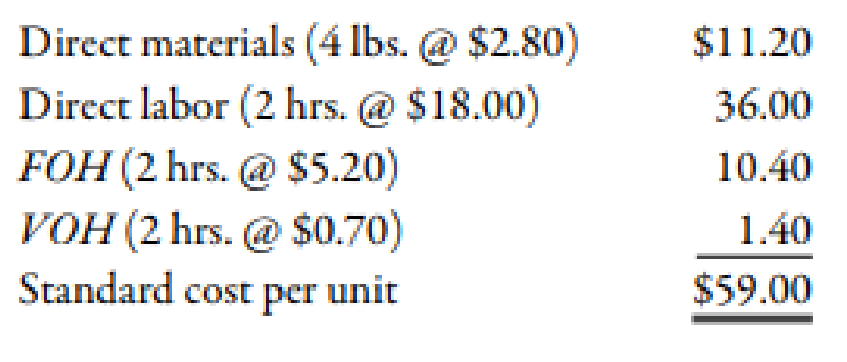Chapter 10, Problem 58E### Managerial Accounting: The Corners...

7th Edition
Maryanne M. Mowen + 2 others
ISBN: 9781337115773

#### Solutions

Chapter
Section### Managerial Accounting: The Corners...

7th Edition
Maryanne M. Mowen + 2 others
ISBN: 9781337115773
Textbook Problem
1 views

# At the beginning of the year, Lopez Company had the following standard cost sheet for one of its chemical products:Lopez computes its overhead rates using practical volume, which is 80,000 units. The actual results for the year are as follows: (a) Units produced: 79,600; (b) Direct labor: 158,900 hours at $18.10; (c) FOH:$831,000; and (d) VOH: $112,400.Required: 1. Compute the variable overhead spending and efficiency variances. 2. Compute the fixed overhead spending and volume variances. 1. To determine Calculate the value of variable overhead spending and efficiency variances. Explanation Variance: The amount obtained when actual cost is deducted from budgeted cost is known as variance. Variance is calculated to find whether the cost is over applied or under applied. Use the following formula to calculate overhead spending variance with the help of columnar approach: Overhead Spending Variance=[Actual Overhead(Actual Hours×Standard Variable Overhead)] Substitute$112,400 for actual overhead, $0.70 for standard variable overhead and 158,900 for actual hours in the above formula. Overhead Spending Variance=$112,400(0.70×158,900)=$1,170(U) Therefore, the overhead spending variance is$1,170 (U).

Use the following formula to calculate efficiency variance:

Substitute 158,900 hours for actual hours, \$0

2.

To determine

Calculate the value of fixed overhead spending and volume variances.

### Still sussing out bartleby?

Check out a sample textbook solution.

See a sample solution

#### The Solution to Your Study Problems

Bartleby provides explanations to thousands of textbook problems written by our experts, many with advanced degrees!

Get Started